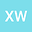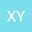••This paper deals with homogeneous Dirichlet boundary value problem to a class of semi-linear equations with p-Laplacian viscoelastic term $$\frac{\partial u}{\partial t}-\Delta u+\int_{0}^{t}g(t-s)\Delta_{p}u(x,s)\mbox{d}s=\mid u\mid^{q(x)-2}u,~~~~~x\in\Omega,~~t\geq 0,$$ the bounded domain $\Omega\subset R^{N}~(N\geq 1)$ with a smooth boundary. We prove that the weak solutions of the above problems blow up in finite time for all $q^{-}> 2k$(~$k$~is defined in $(2.3)$), when the initial energy is positive and the function~$g$~satisfies suitable conditions. This result generalized and improved the result by S.~A.~Messaoudi~\cite{1}.